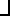# Maths - Clifford Algebra - Inner Products

There are different difinitions of the inner product as follows:

 • Dot product •H Hestines inner product. Like the dot product except that it is zero whenever one of its arguments is a scalar.left contraction inner productright contraction inner product

These all give the same result when the operands are vectors, which means we could substitute any of them in the usual equations:

 a•b = ½ (ab + ba) This is symmetrical (a•b = b•a) a^b = ½ (ab - ba) This is anti-symmetrical (a^b = - b^a) a * b = a•b + a^b

Where:

• a and b are vectors
• Kk a multivector of grade k

We can extend this to the multipication of a vector by a general multivector as follows:

a•K = ½ (aK + (-1)k+1Ka)

a^K = ½ (aK + (-1)k Ka)

a*K = a•K + a^K

Where k is the grade of K. The (-1)k factor alternates the sign as follows:

 grade k (-1)k (-1)k+1 a•K = ½ (aK + (-1)k+1Ka) 0 (scalar) 1 -1 a•K = ½ (aK - Ka) = 0 1 (vector) -1 1 a•K = ½ (aK + Ka) = aK 2 (bivector) 1 -1 a•K = ½ (aK - Ka) = 0 3 (trivector) -1 1 a•K = ½ (aK + Ka) = aK

Where I can, I have put links to Amazon for books that are relevant to the subject, click on the appropriate country flag to get more details of the book or to buy it from them.Clifford Algebra to Geometric Calculus: A Unified Language for Mathematics and Physics (Fundamental Theories of Physics). This book is intended for mathematicians and physicists rather than programmers, it is very theoretical. It covers the algebra and calculus of multivectors of any dimension and is not specific to 3D modelling.New Foundations for Classical Mechanics (Fundamental Theories of Physics). This is very good on the geometric interpretation of this algebra. It has lots of insights into the mechanics of solid bodies. I still cant work out if the position, velocity, etc. of solid bodies can be represented by a 3D multivector or if 4 or 5D multivectors are required to represent translation and rotation.